Home > Blog >

### How to calculate the number of pulses?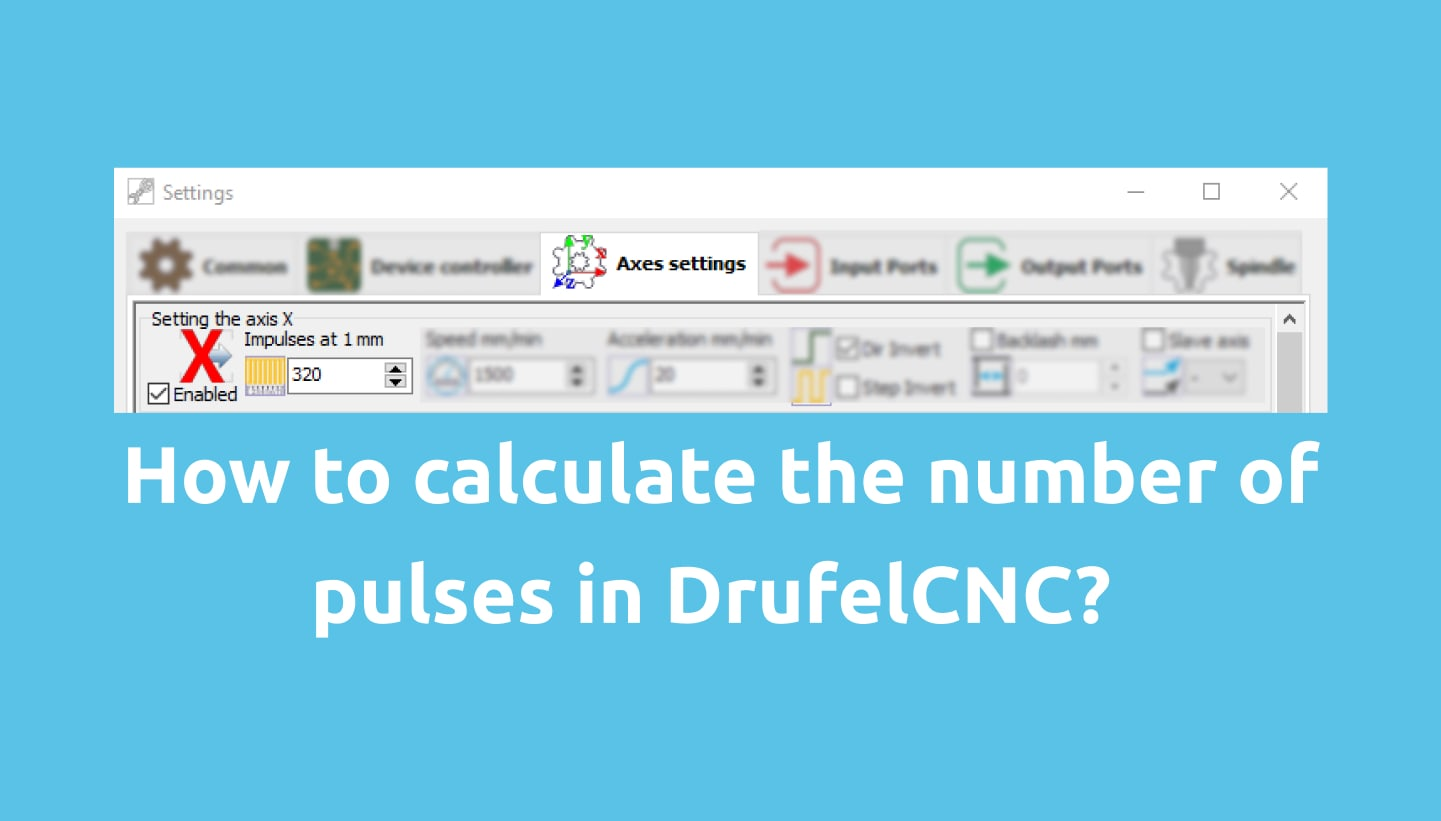You can calculate the number of pulses yourself. We have prepared detailed step-by-step instructions on how to calculate the number of pulses.
1. Open DrufelCNC.
2. Go to the settings window.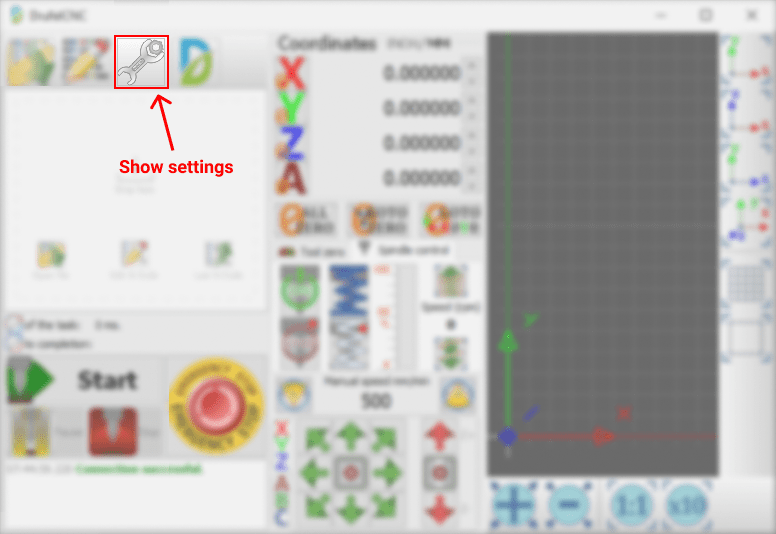3. In the settings window, set 320 pulses at 1 mm.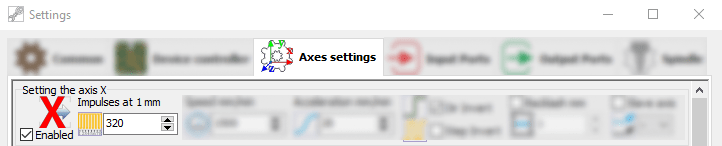4. Save the settings. Go to the main window. Set the values of all coordinates to zero by clicking the ALL ZERO button.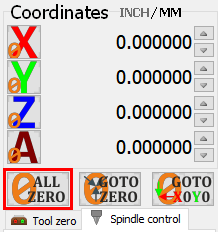5. Go to the "Edit G code" window.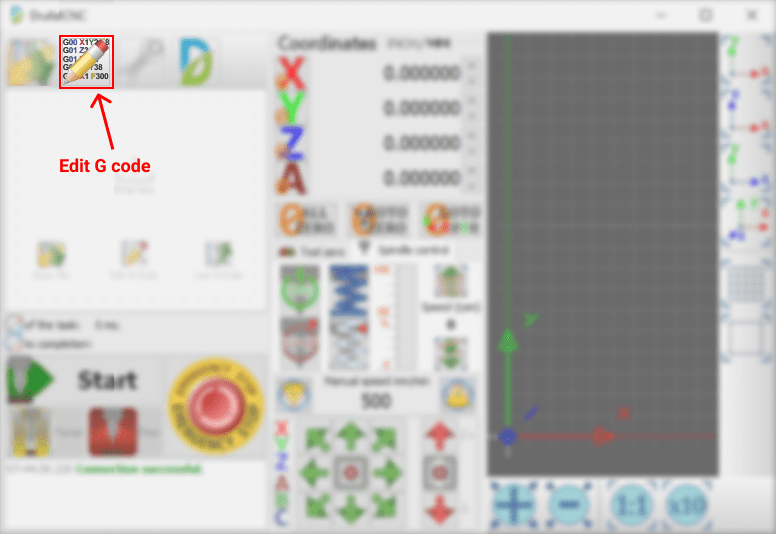6. Enter the following g-code: G01 X50.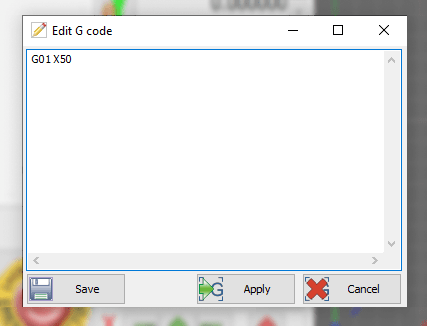This g-code moves the tool by 50 millimeters, with the correct setting of the number of pulses by 1 mm.

7. Start the execution of the g-code by clicking the Start button.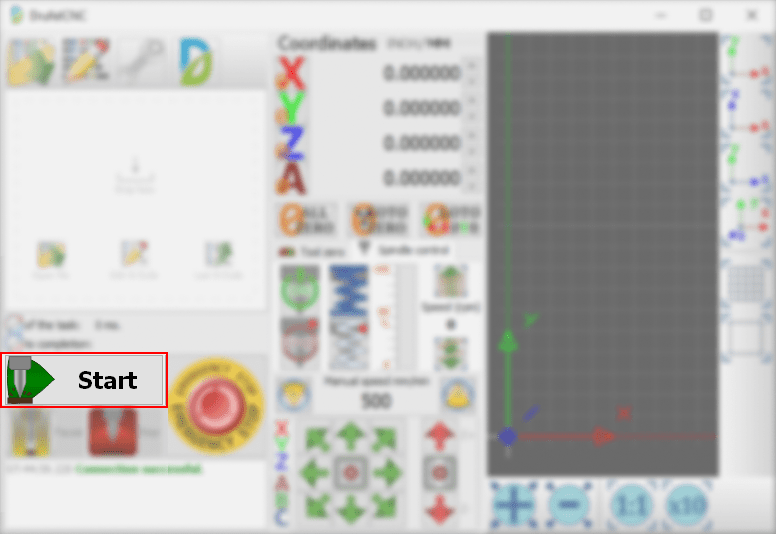8. After moving the tool, measure the length from the start point to the end point of the move.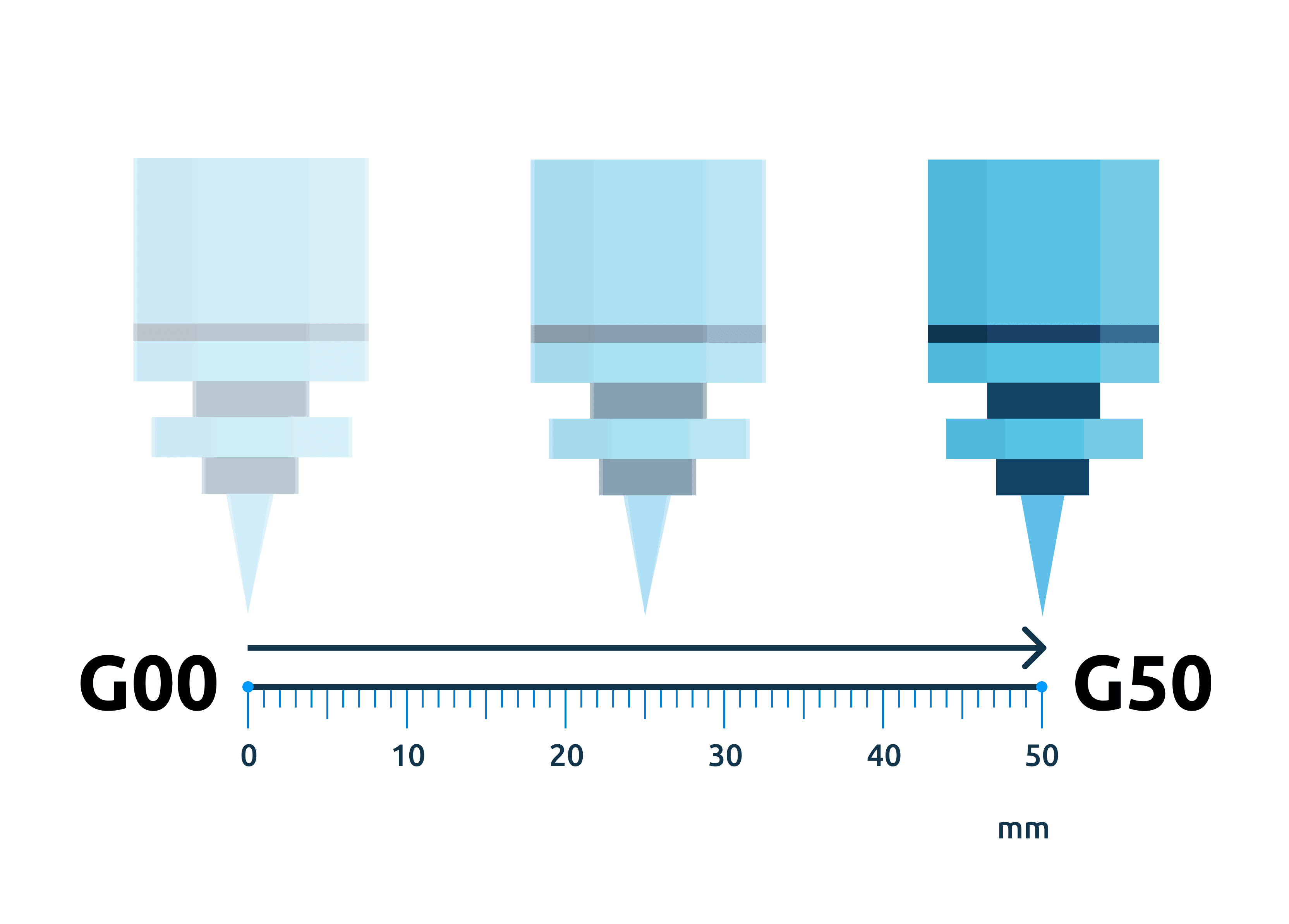Let's calculate the required number of pulses using the formula:
number of pulses * length of movement in a predetermined g-code / the actual length of displacement

For example:
320 * 50 / х
х = length

1. Let's set new values:
If the tool has moved 100 mm, then
320 * 50/100 = 160

So you need to set 160 pulses to 1 mm.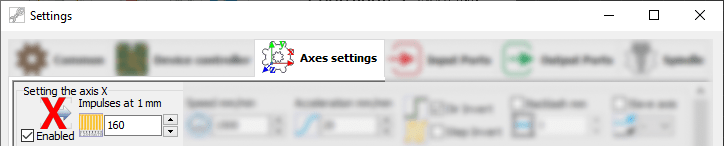2. Let's set new values:
If the tool has moved 25 mm, then
320 * 50/25 = 640

So you need to set 640 pulses to 1 mm.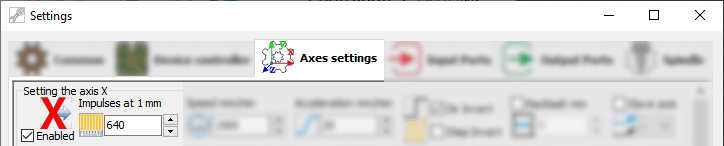3. Let's set new values:
If the tool has moved 38 mm, then
320 * 50/38 = 486,4

So you need to set 486,4 pulses to 1 mm.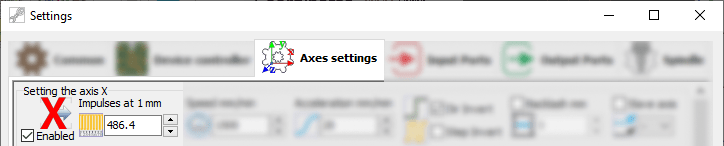Result: The number of impulses per 1 mm after the calculations can increase or decrease. Also, the number may not be whole.

You can find out how to set up a stepper motor driver here.